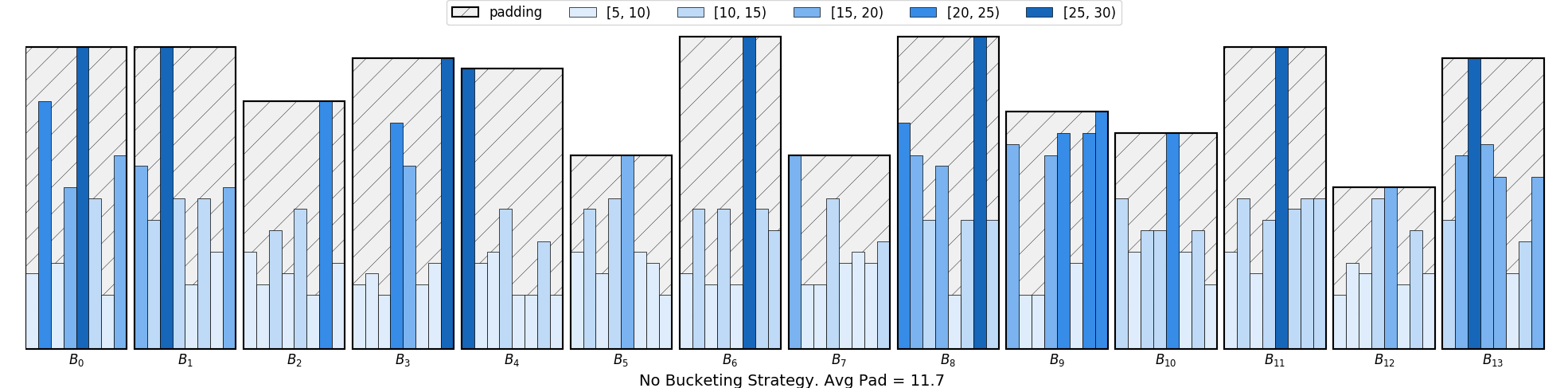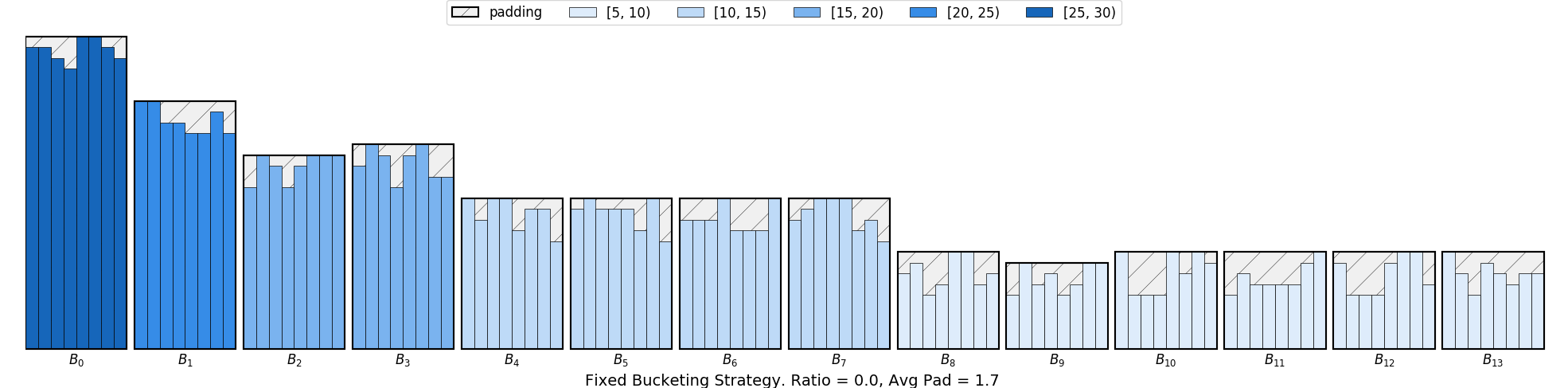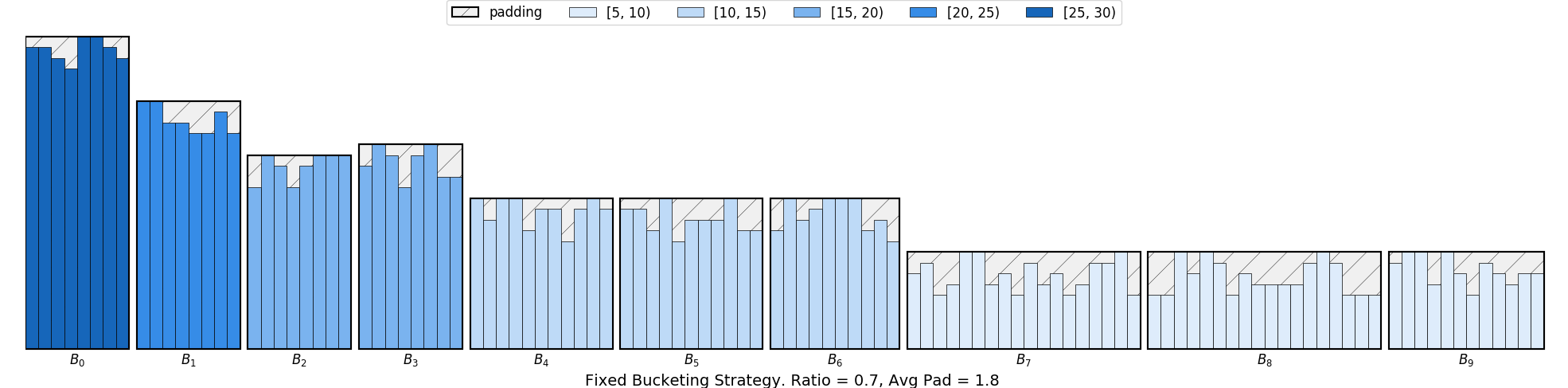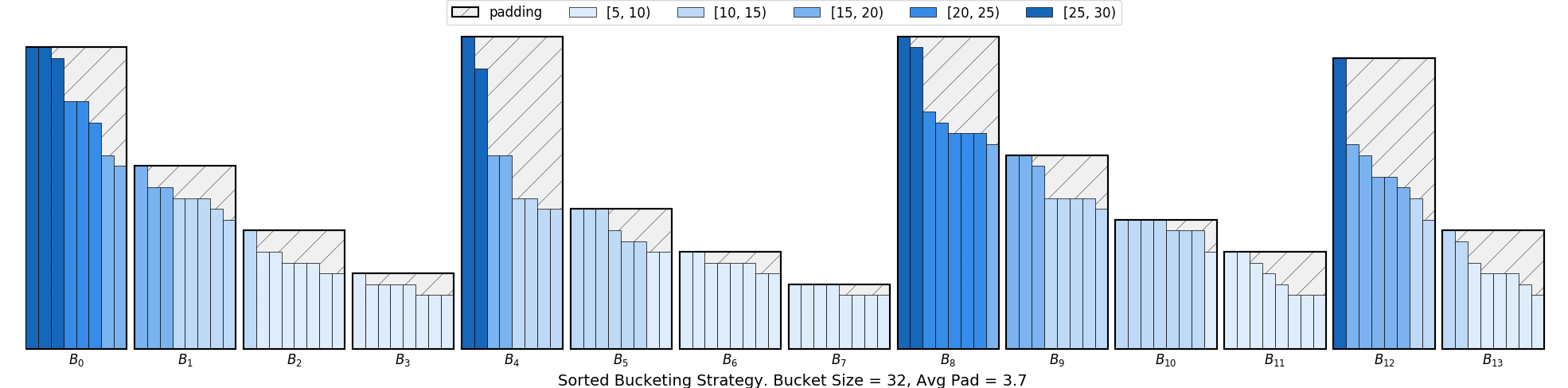In this tutorial, we show how to load and process the sentiment dataset to form batches that can be processed efficiently, using classes from gluonnlp.data.sampler and gluonnlp.data.batchify. We use IMDB dataset as an example, where the dataset has 50,000 movie reviews, labeled as positive or negative. The dataset is split into the training/testing dataset, each consisting of 25,000 reviews.

Let us see a quick example.

>>> import mxnet as mx
>>> from mxnet import gluon, nd
>>> import gluonnlp as nlp

>>> train_dataset, test_dataset = [nlp.data.IMDB(root='data/imdb', segment=segment)
>>>                                for segment in ('train', 'test')]

>>> print('#training samples={:d}, #testing samples={:d}'.format(len(train_dataset),
>>>                                                              len(test_dataset)))

#training samples: 25000, #testing samples: 25000

>>> print(train_dataset)

['Bromwell High is a cartoon comedy. It ran at the same time as some other programs
about school life, such as "Teachers". My 35 years in the teaching profession lead
me to believe that Bromwell High\'s satire is much closer to reality than is "Teachers".
The scramble to survive financially, the insightful students who can see right through
their pathetic teachers\' pomp, the pettiness of the whole situation, all remind me of
the schools I knew and their students. When I saw the episode in which a student repeatedly
tried to burn down the school, I immediately recalled ......... at .......... High. A
classic line: INSPECTOR: I\'m here to sack one of your teachers. STUDENT: Welcome to
Bromwell High. I expect that many adults of my age think that Bromwell High is far fetched.
What a pity that it isn\'t!', 9]


In the above example, we load train_dataset and test_dataset, which are both SimpleDataset objects.

SimpleDataset: wrapper for lists and arrays. Each entry in the train_dataset is a [string, score] pair, where the score falls into [1, 2, …, 10]. Thus in the given example, 9 indicates a positive feedback on the movie.

## Data Processing¶

The next step is to preprocess the data so that it can be used to train the model. The following code shows how to tokenize the string with SpacyTokenizer and then clip the list of output tokens by length.

>>> tokenizer = nlp.data.SpacyTokenizer('en')
>>> # We use 50 as maximum length for illustration
>>> # For actual learning, we may use a large value such as 500
>>> length_clip = nlp.data.ClipSequence(50)
>>> seq, score = train_dataset
>>> print(length_clip(tokenizer(seq)))

['Bromwell', 'High', 'is', 'a', 'cartoon', 'comedy', '.', 'It', 'ran', 'at', 'the', 'same',
'time', 'as', 'some', 'other', 'programs', 'about', 'school', 'life', ',', 'such', 'as',
'"', 'Teachers', '"', '.', 'My', '35', 'years', 'in', 'the', 'teaching', 'profession', 'lead',
'me', 'to', 'believe', 'that', 'Bromwell', 'High', "'s", 'satire', 'is', 'much', 'closer',
'to', 'reality', 'than', 'is']


Now, we are ready to preprocess the whole dataset. The following code shows how to tokenize the dataset parallelly.

>>> import time
>>> import multiprocessing as mp
>>> length_clip = nlp.data.ClipSequence(500)

>>> # Dataset preprocessing
>>> def preprocess(x):
>>>     data, label = x
>>>     # In the labeled train/test sets, a negative review has a score <= 4
>>>     # out of 10, and a positive review has a score >= 7 out of 10. Thus
>>>     # reviews with more neutral ratings are not included in the train/test
>>>     # sets. We labeled a negative review whose score <= 4 as 0, and a
>>>     # positive review whose score >= 7 as 1. As the neural ratings are not
>>>     # included in the datasets, we can simply use 5 as our threshold.
>>>     label = int(label > 5)
>>>     data = length_clip(tokenizer(data))
>>>     return data, label
>>>
>>> def get_length(x):
>>>     return float(len(x))
>>>
>>> def preprocess_dataset(dataset):
>>>     start = time.time()
>>>     pool = mp.Pool()
>>>     dataset = gluon.data.SimpleDataset(pool.map(preprocess, dataset))
>>>     lengths = gluon.data.SimpleDataset(pool.map(get_length, dataset))
>>>     end = time.time()
>>>     print('Done! Tokenizing Time={:.2f}s, #Sentences={}'.format(end - start, len(dataset)))
>>>     return dataset, lengths
>>>
>>> # Preprocess the dataset
>>> train_dataset, train_data_lengths = preprocess_dataset(train_dataset)
>>> test_dataset, test_data_lengths = preprocess_dataset(test_dataset)

Tokenize using spaCy...

Done! Tokenizing Time=12.85s, #Sentences=25000

Done! Tokenizing Time=12.99s, #Sentences=25000


Then, we are going to construct a vocabulary for the training dataset. The vocabulary will be used to convert the tokens to numerical indices, which facilitates the creation of word embedding matrices.

>>> import itertools
>>> train_seqs = [sample for sample in train_dataset]
>>> counter = nlp.data.count_tokens(list(itertools.chain.from_iterable(train_seqs)))
>>> vocab = nlp.Vocab(counter, max_size=10000, padding_token=None,
>>>                   bos_token=None, eos_token=None)
>>> print(vocab)

Vocab(size=10001, unk="<unk>", reserved="None")

>>> # Convert string token to its index in the dictionary
>>> def token_to_idx(x):
>>>     return vocab[x], x
>>>
>>> pool = mp.Pool()
>>> train_dataset = pool.map(token_to_idx, train_dataset)
>>> test_dataset = pool.map(token_to_idx, test_dataset)
>>> pool.close()
>>> print(train_dataset[:50])

[0, 2012, 8, 4, 1116, 231, 3, 51, 2311, 40, 1, 188, 67, 20, 59, 97, 6190, 49, 422, 133,
2, 160, 20, 13, 0, 13, 3, 374, 5063, 174, 9, 1, 5390, 6674, 498, 83, 7, 282, 12, 0, 2012,
15, 2042, 8, 88, 2661, 7, 714, 87, 8]


The next step is to construct a dataloader for training. As the sequences have variable lengths, we need to pad the sequences so that they have the same lengths in the minibatch, which allows the fast tensor manipulation in GPU.

>>> batchify_fn = nlp.data.batchify.Tuple(nlp.data.batchify.Pad(axis=0, pad_val=0),
>>>                                       nlp.data.batchify.Stack())


Tuple wraps multiple batchify functions and applies each input function on each input field, respectively. In this case, we are applying Pad on the sequence and Stack on the labels. Given the batchify function, we can construct the dataloaders for both training samples and testing samples.

>>> train_dataloader = gluon.data.DataLoader(dataset=train_dataset,
>>>                                          batch_size=batch_size,
>>>                                          shuffle=True,
>>>                                          batchify_fn=batchify_fn)
>>>                                         batch_size=batch_size,
>>>                                         shuffle=False,
>>>                                         batchify_fn=batchify_fn)


As DataLoader is iterable, we can iterate over the dataset easily using the following code:

>>> for data, label in train_dataloader:


In the above example, minibatcheas are formed using uniform sampling, which can cause a large amount of padding as shown in the figure below.In light of this, we consider constructing a sampler using bucketing, which defines how the samples in a dataset will be iterated in a more economic way.

 >>> batch_sampler = nlp.data.sampler.FixedBucketSampler(train_data_lengths,
>>>                                                     batch_size=16,
>>>                                                     num_buckets=10,
>>>                                                     ratio=0,
>>>                                                     shuffle=True)
>>> print(batch_sampler.stats())

FixedBucketSampler:
sample_num=25000, batch_num=1567
key=[68, 116, 164, 212, 260, 308, 356, 404, 452, 500]
cnt=[981, 1958, 5686, 4614, 2813, 2000, 1411, 1129, 844, 3564]
batch_size=[16, 16, 16, 16, 16, 16, 16, 16, 16, 16]


In this example, we use a FixedBucketSampler, which assigns each data sample to a fixed bucket based on its length.

The bucket keys are either given or generated from the input sequence lengths. We construct 10 buckets, where cnt shows the number of samples belonging to each bucket. A graphic illustration of using FixedBucketSampler can be seen as follows:To further improve the throughput, we can consider scaling up the batch size of smaller buckets. This can be achieved by using a parameter ratio. Assume the $$i$$ th key is $$K_i$$ , the default batch size is $$B$$ , the ratio to scale the batch size is $$\alpha$$ and the batch size corresponds to the $$i$$ th bucket is $$B_i$$ . We have:

$B_i = \max(\alpha B \times \frac{\max_j sum(K_j)}{sum(K_i)}, B)$Thus, setting this to a value larger than 0, like 0.5, will scale up the batch size of the smaller buckets.

 >>> batch_sampler = nlp.data.sampler.FixedBucketSampler(train_data_lengths,
>>>                                                     batch_size=16,
>>>                                                     num_buckets=10,
>>>                                                     ratio=0.5,
>>>                                                     shuffle=True)
>>> print(batch_sampler.stats())

FixedBucketSampler:
sample_num=25000, batch_num=1306
key=[68, 116, 164, 212, 260, 308, 356, 404, 452, 500]
cnt=[981, 1958, 5686, 4614, 2813, 2000, 1411, 1129, 844, 3564]
batch_size=[58, 34, 24, 18, 16, 16, 16, 16, 16, 16]


Now, we can create dataloader using bucketing sampler for both training set.

>>> train_dataloader = gluon.data.DataLoader(dataset=train_dataset,
>>>                                          batch_sampler=batch_sampler,
>>>                                          batchify_fn=batchify_fn)


In our sampler API, we also provide another sampler called SortedBucketSampler, which results in the following padding pattern:With this strategy, we partition data to a number of buckets with size batch_size * mult, where mult is a multiplier to determine the bucket size. Each bucket contains batch_size * mult elements. The samples inside each bucket are sorted based on sort_key and then batched.

>>> batch_sampler = nlp.data.sampler.SortedBucketSampler(train_data_lengths,
>>>                                                     batch_size=16,
>>>                                                     mult=100,
>>>                                                     shuffle=True)


More details about the training using pre-trained language model and bucketing can be found in the sentiment analysis tutorial.